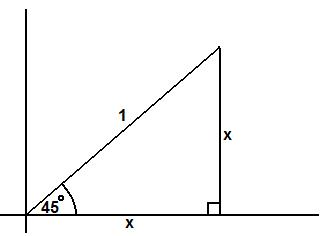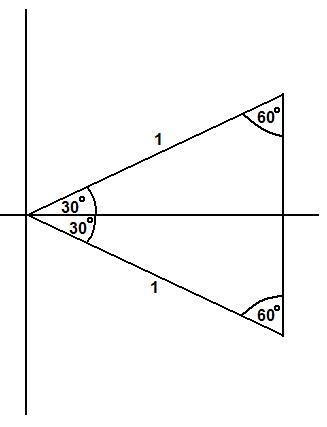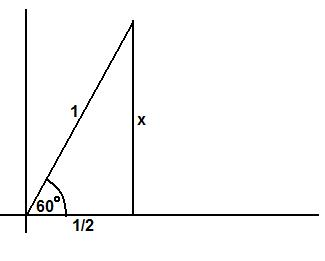#### You may also like### Some(?) of the Parts

A circle touches the lines OA, OB and AB where OA and OB are perpendicular. Show that the diameter of the circle is equal to the perimeter of the triangleA 1 metre cube has one face on the ground and one face against a wall. A 4 metre ladder leans against the wall and just touches the cube. How high is the top of the ladder above the ground?### At a Glance

The area of a regular pentagon looks about twice as a big as the pentangle star drawn within it. Is it?

# Where Is the Dot?

##### Age 14 to 16Challenge Level

The first part of this problem was answered correctly by a number of people. As the film suggests, we can view the dot as a vertex on a right angled triangle whose hypotenuse has length one. The angle $45^{\circ}$ is special because at this point the triangle is equalateral. Let $x$ denote the height of the dot after it has turned through $45^{\circ}$.Pythagoras' theorem states that $x^2+x^2=1^2$, i.e. $x^2=\frac{1}{2}$ so $x=\frac{1}{\sqrt{2}}$.

The next part of the question asked for similar ways of calculating the height of the dot after it had turned through $30^{\circ}$ and $60^{\circ}$.

Consider the right angled triangle we obtain after turning through $30^{\circ}$. If we reflect this triangle in the horizontal axis we obtain an equalateral triangle with sides of length $1$ as shown.This implies that the height of the dot must be $\frac{1}{2}$. We deduce that at $60^{\circ}$ we end up with the following triangle:By Pythagoras, the height of the dot must satisfy the equation $x^2+(\frac{1}{2})^2=1^2$ which implies that $x=\sqrt{1-\frac{1}{4}}=\sqrt{\frac{3}{4}}=\frac{\sqrt{3}}{2}$.

By symmetry, now that we know the height of the dot for angles of $30^{\circ}$, $45^{\circ}$ and $60^{\circ}$, we can state the height for angles which are a multiple of $30^{\circ}$, $45^{\circ}$ or $60^{\circ}$. See if you can list these angles and the corresponding heights of the dot.